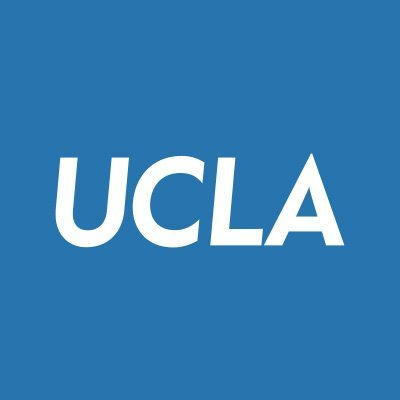# Introduction to Data Analysis using MATLAB.

Organisation
UCL
Start - End
11 Oct - 12 Oct
Study Options
Remote
Fee
£ 200

This course aims to introduce delegates to the MATLAB programming language for data analysis; begins with a brief overview of the MATLAB interface, then moves on to importing data, statistical analysis, graphical representation, and inbuilt MATLAB applications.

This course aims to introduce delegates to the MATLAB programming language for data analysis; begins with a brief overview of the MATLAB interface, then moves on to importing data, statistical analysis, graphical representation, and inbuilt MATLAB applications. While no prior knowledge of MATLAB programming is required, it is essential that delegates have a basic understanding of statistical concepts such as summary statistics, p-values, confidence intervals and simple regression.

This course covers the following topics:

• Getting started with the MATLAB interface
• Basic MATLAB commands: objects, functions, help and operations
• Creating and importing data in MATLAB
• Basic data manipulation in MATLAB
• Descriptive statistics
• Graphs
• Significance tests
• Overview of MATLAB applications
• Bootstrapping (time permitting)
• Regression Analysis (time permitting)

Please note: this is not a statistical theory course, it is intended to teach delegates about the MATLAB software. As such, dedicated time cannot be given to describing and explaining theory and statistical tests (these are taught on our course - 'Introduction to research methods and statistics').